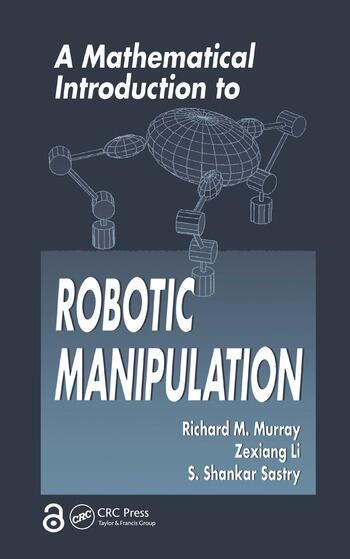# A Mathematical Introduction to Robotic Manipulation

## 1st Edition

Richard M. Murray

CRC Press
Published March 22, 1994
Textbook - 480 Pages
ISBN 9780849379819 - CAT# 7981

For Instructors Request Inspection Copy

was \$145.00

USD\$116.00

SAVE ~\$29.00

FREE Standard Shipping!

## Preview

### Summary

A Mathematical Introduction to Robotic Manipulation presents a mathematical formulation of the kinematics, dynamics, and control of robot manipulators. It uses an elegant set of mathematical tools that emphasizes the geometry of robot motion and allows a large class of robotic manipulation problems to be analyzed within a unified framework.

The foundation of the book is a derivation of robot kinematics using the product of the exponentials formula. The authors explore the kinematics of open-chain manipulators and multifingered robot hands, present an analysis of the dynamics and control of robot systems, discuss the specification and control of internal forces and internal motions, and address the implications of the nonholonomic nature of rolling contact are addressed, as well.

The wealth of information, numerous examples, and exercises make A Mathematical Introduction to Robotic Manipulation valuable as both a reference for robotics researchers and a text for students in advanced robotics courses.

#### Instructors

We provide complimentary e-inspection copies of primary textbooks to instructors considering our books for course adoption.